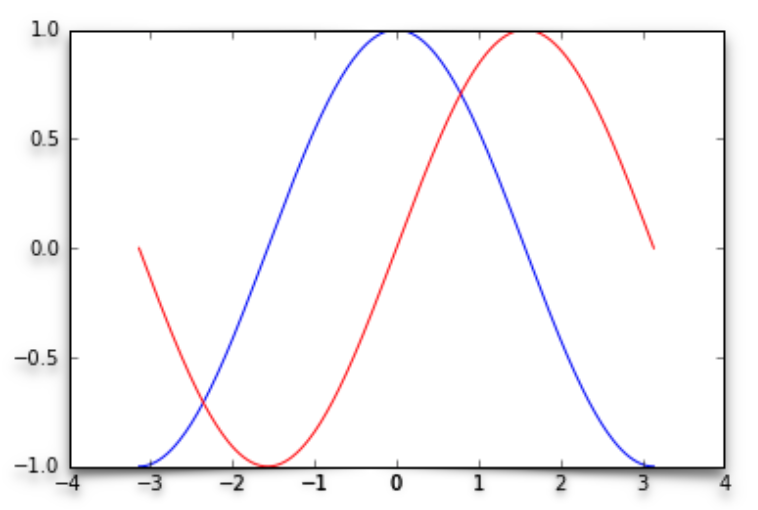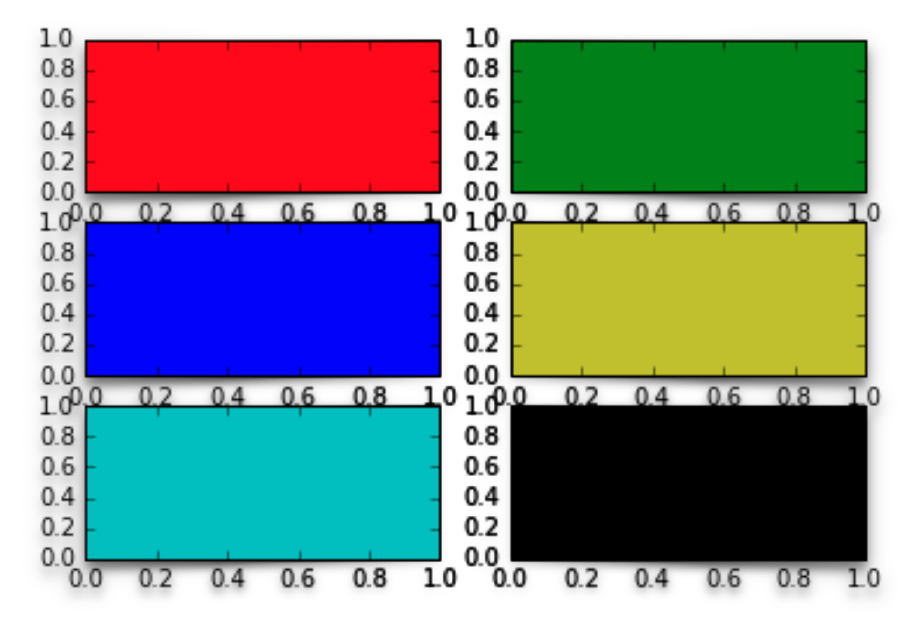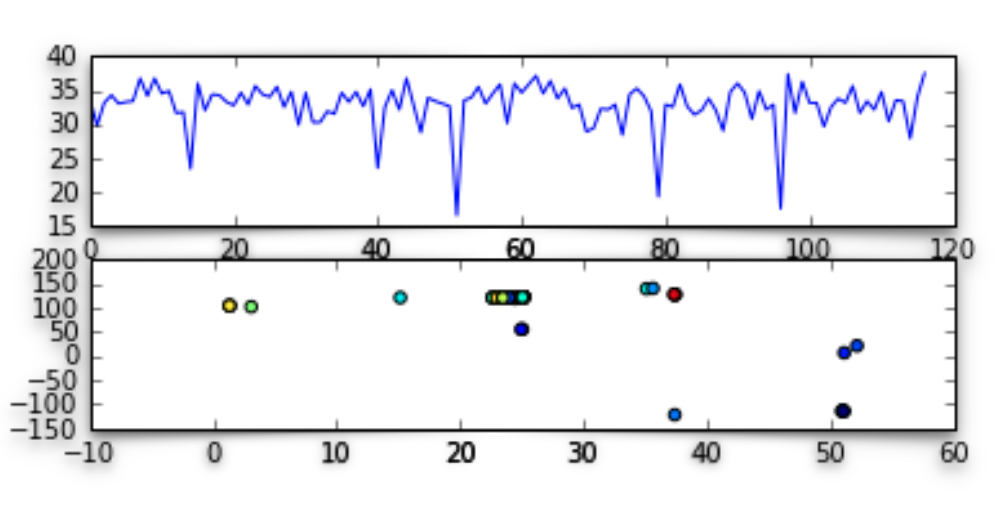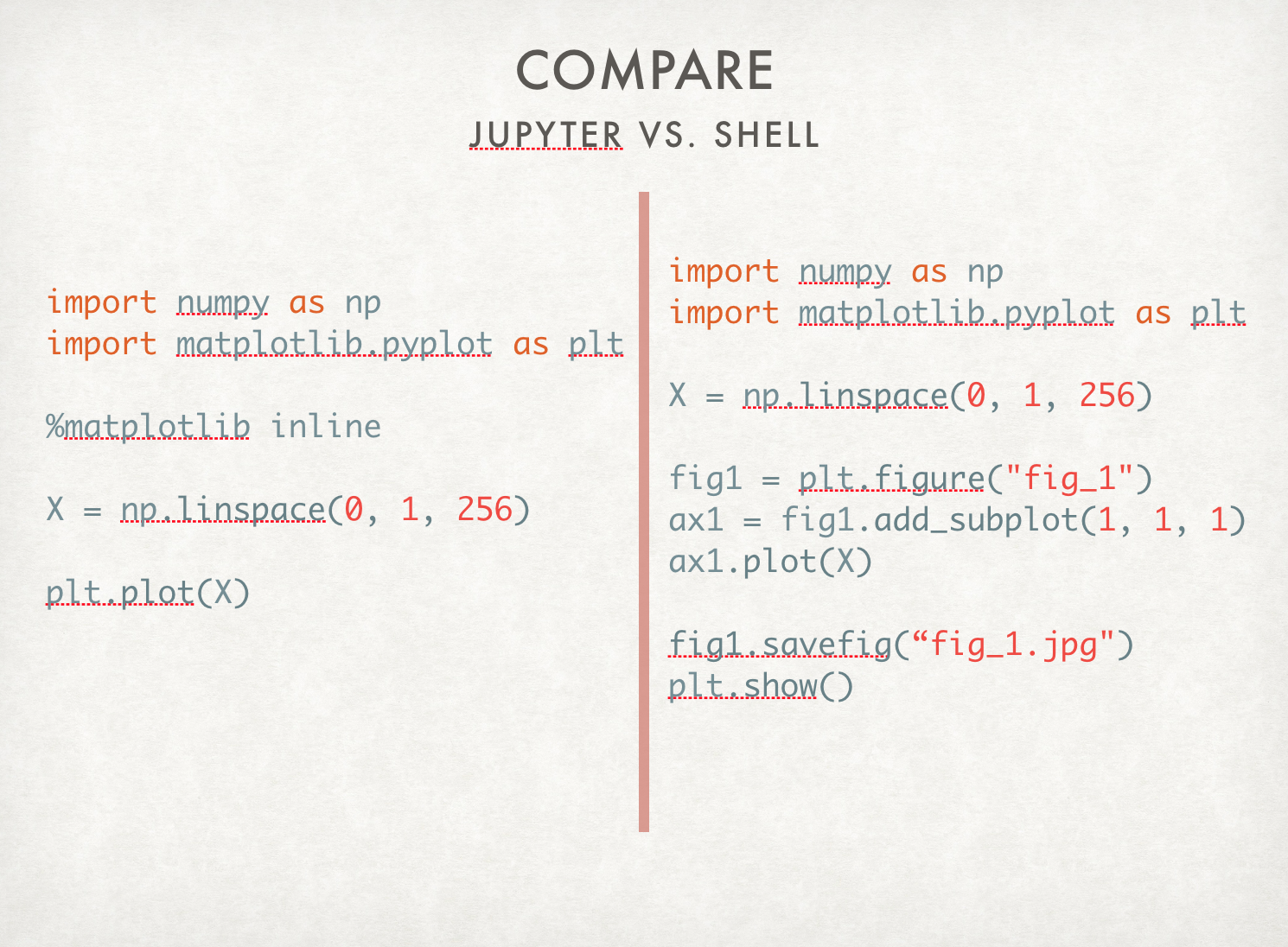# Matplotlib Introduction and Using

- 1 min

This slides is made for Taipei.py meetup. In this talk, I gave base knowledge how to use Matplotlib and gave some exercises in order to let readers understand what and why they need to know this knowledge before applying it.

### What is Matplotlib?

Matplotlib is a tool that implemented in Python. The primary target of this tool is another replacement of Matlab. Matplotlib has a convenient command-line interface. You can also trigger IPython to fast plot. You can use OO interface to compose complex figures.

• Pros

• You can directly use Python to manipulate data and tweak.
• Multiplot is better in Matplotlib.
• You can apply LaTex to render plot.
• Cons

• Because of the two interfaces (OO and GUI) exist, API is more complicated. You would see there are two or more methods to compose the same figures.

• Official document is hard to read.### Summary

In Matplotlib, class is following the rule Figure -> Axes -> (Line2D, Text, etc). One Figure can contain several Axes. Use Axes in Matplotlib indicates a plotting area.

For more details, you can check my slides.